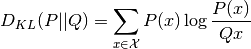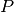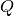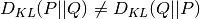Shortcuts

# KL Divergence¶

## Module Interface¶

class torchmetrics.KLDivergence(log_prob=False, reduction='mean', **kwargs)[source]

Computes the KL divergence:Whereandare probability distributions whereusually represents a distribution over data andis often a prior or approximation of. It should be noted that the KL divergence is a non-symetrical metric i.e..

As input to forward and update the metric accepts the following input:

As output of forward and compute the metric returns the following output:

Parameters
• log_prob (bool) – bool indicating if input is log-probabilities or probabilities. If given as probabilities, will normalize to make sure the distributes sum to 1.

• reduction (Literal[‘mean’, ‘sum’, ‘none’, None]) –

Determines how to reduce over the N/batch dimension:

• 'mean' [default]: Averages score across samples

• 'sum': Sum score across samples

• 'none' or None: Returns score per sample

• kwargs (Any) – Additional keyword arguments, see Advanced metric settings for more info.

Raises
• TypeError – If log_prob is not an bool.

• ValueError – If reduction is not one of 'mean', 'sum', 'none' or None.

Note

Half precision is only support on GPU for this metric

Example

>>> import torch
>>> from torchmetrics.functional import kl_divergence
>>> p = torch.tensor([[0.36, 0.48, 0.16]])
>>> q = torch.tensor([[1/3, 1/3, 1/3]])
>>> kl_divergence(p, q)
tensor(0.0853)


Initializes internal Module state, shared by both nn.Module and ScriptModule.

## Functional Interface¶

torchmetrics.functional.kl_divergence(p, q, log_prob=False, reduction='mean')[source]

Computes KL divergenceWhereandare probability distributions whereusually represents a distribution over data andis often a prior or approximation of. It should be noted that the KL divergence is a non-symetrical metric i.e..

Parameters
• p (Tensor) – data distribution with shape [N, d]

• q (Tensor) – prior or approximate distribution with shape [N, d]

• log_prob (bool) – bool indicating if input is log-probabilities or probabilities. If given as probabilities, will normalize to make sure the distributes sum to 1

• reduction (Literal[‘mean’, ‘sum’, ‘none’, None]) –

Determines how to reduce over the N/batch dimension:

• 'mean' [default]: Averages score across samples

• 'sum': Sum score across samples

• 'none' or None: Returns score per sample

Example

>>> import torch
>>> p = torch.tensor([[0.36, 0.48, 0.16]])
>>> q = torch.tensor([[1/3, 1/3, 1/3]])
>>> kl_divergence(p, q)
tensor(0.0853)

Return type

Tensor

© Copyright Copyright (c) 2020-2023, Lightning-AI et al... Revision 825d17f3.

Built with Sphinx using a theme provided by Read the Docs.
Versions
latest
stable
v0.11.3
v0.11.2
v0.11.1
v0.11.0
v0.10.3
v0.10.2
v0.10.1
v0.10.0
v0.9.3
v0.9.2
v0.9.1
v0.9.0
v0.8.2
v0.8.1
v0.8.0
v0.7.3
v0.7.2
v0.7.1
v0.7.0
v0.6.2
v0.6.1
v0.6.0
v0.5.1
v0.5.0
v0.4.1
v0.4.0
v0.3.2
v0.3.1
v0.3.0
v0.2.0
v0.1.0